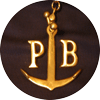Phil's Boat

 Mission Progress The Boat Control Software Map

Software

This is written using the Arduino version of C, and libraries written for Arduino controllers.

To be honest there isn't much that is clever about the software, however there are a couple of things worth sharing.

The first is the trigonometry calculations, without using the standard library. The second is how to run the Arduino in a low-power mode.

Trigonometry

Although the exact heading to the next way point is not necessary (because the boat won't steer exactly where you want it anyway), the boat does want to head in roughly the right direction. In practice it is pretty easy to get the heading within a degree or so, which is probably much better than the ability of the boat to follow a heading.

To calculate the desired heading from the current position, and the next way-point uses both cos(theta) and arctan(a/b) (see below why I need this). This would be easy if I just used the relevant Arduino library, but am concerned that I may run out of program memory, and since I don't need very good accuracy, I thought I'd investigate other methods.

The current position is obtained from the GPS unit as degrees lattitude and longitude. To calculate the desired heading, I first calculate the North-South distance to go, and also the East-West distance. Then I can calculate the angle to head. Calculating the distance in the north-south direction is very easy as distance across the surface of the ocean is simply the difference in lattitude mutiplied by a constant. The constant is the circumference of the earth divided by 360º. It is about 67 miles per degree, or 111km per degree. The circumference of the earth is about 24,000 miles, or 40,000km. The latter was how Napolean decided on the length of the metre, ie 1/10,000,000 of the distance between the poles and the equator.

The east-west distance is not so easy, as the distance between lines of longitude decreases away from the equator (and towards the poles). So to know the distance in miles between where you are and where you want to be, you need to allow for this variation. The multiplier between degrees of longitude and miles decreases by cos(theta), where theta is the degrees of lattitude. At the equator the scaling factor is the same as for lattitude, ie 67 miles per degree. At 60º north for example the scaling is half of this. So the mathematical formula for east-west distance is: 67 * cos(lattitude) miles per degree. Therefore the system needs to be able to calculate cos(theta). The obvious method to calculate cos(theta) is to use a few terms in the Maclaurin expansion. This is very simple, and is cos(x) = 1-x2/2+x4/24 and so on (note that x has to be in radians: 360 degrees = 2?, ie 2 times pi or 6.28; so 1 radian is about 52 degrees). Taking advantage of the (hopeful) fact that the boat won't get too close to the poles, only a very few terms are needed to get an accuracy of better than needed. In practice, only the x-squared term is needed to get better than 1% accuracy up to a lattitude of 55º, however I opted for the x4 term as well, giving better than 0.2% up to 55º.

The bearing is the angle of the line that is the hypotenuse of the right-angled triangle created by the north-south and the east-west distances. If N is the north-south distance, and E is the east-west distance, then the angle needed is arctan(N/E), which is in radians. So the bearing is 360/2/?*arctan(N/E). For the reasons of memory saving described above, I wanted an easy mathematical way to calculate arctan(), approximately (to better than 1 degree).  Looking at a graph of arctan(),  you can see that  between 0 and 45º, the graph is reasonably straight, but from 45º to 90º it is much more curved. After that it repeats. However,  I can take advantage that by swopping the N and E dimensions, I am effectively drawing the same triangle rotated by 90º, and so the angle I care about can always be less than 45º.
(In more algebraic terms, arctan(N/E) = 90º-arctan(E/N).) So I really only need a way of calculating arctan() that is OK up to 45º and don't care if is badly inaccurate from 45º to 90º. I can also change the sign of the N and E distances to allow for the boat going south (when N is actually negative) instead of north for example. Back to arctan(): since it is a pretty smooth curve up to 45º, I thought a simple quadratic polynomial might do (something like: bearing angle = a+b(N/E) + c(N/E)2). With a quick bit of work using Excel solver, and forcing the values so that it gives the correct values at 0 and 45º, a=0 (this is obvious), b=60.7974, and c = -15.7974. Using this gives a worst error of less than one-quarter of a degree from zero to 45º: perfectly good enough for the boat.

So that's how the heading calculations are done without the trignometry library.

If you look here, there is a very nice, simple way of calculating arctan from the lengths of the two sides. Unfortunately, it requires the length of the hypotenuse of the triangle to be 1, which would mean calculating the square root of a number at some point. This isn't very easy either, so I didn't use this.

Low Power Operation

To be continued...